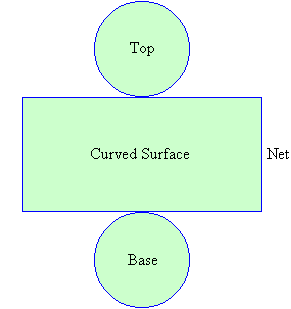Year 8 Interactive Maths - Second Edition

## Cones and Cylinders

Cones and cylinders have curved surfaces as shown below.  So, they are not prisms or polyhedra.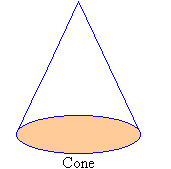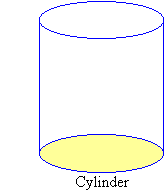### Cones

If one end of a line is rotated about a second fixed line while keeping the line's other end fixed, then a cone is formed.  The point about which the line is rotated is called the vertex and the base of the cone is a circle.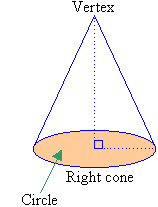A cone is said to be right when the vertex is directly above the centre of the base.

The net of a three-dimensional object is a representation of its faces in two dimensions.

The net of a cone consists of the following two parts:

• a circle that gives the base; and
• a sector that gives the curved surface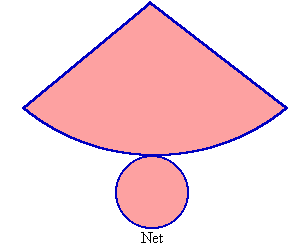### Cylinders

If a line is rotated about a second fixed line while keeping both lines parallel, then a cylinder is formed.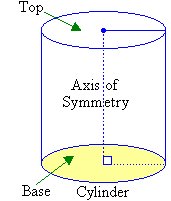###### Note:
• The fixed line about which the line is rotated is called the axis of symmetry.
• The base of the cylinder is a circle and the top of the cylinder is also a circle.
• A cylinder is said to be right when the line joining the centre of the base and the centre of the top is perpendicular to the base of the cylinder.
• The cross-sections parallel to the base are circles and are all identical.

The net of a cylinder consists of three parts:

• One circle gives the base and another circle gives the top.
• A rectangle gives the curved surface.